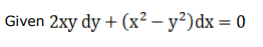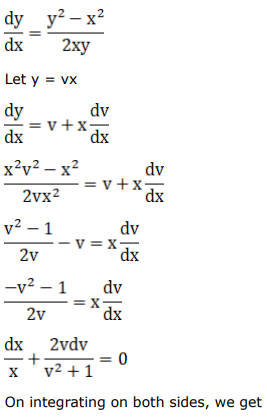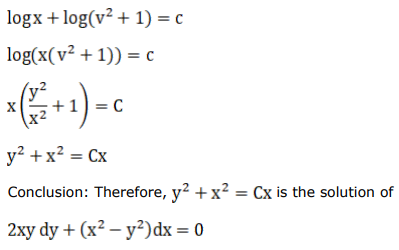# Mark against the correct answer in the following:

Question:

Mark $(\sqrt{)}$ against the correct answer in the following:

The general solution of the DE $2 x y d y+\left(x^{2}-y^{2}\right) d x=0$ is

A. $x^{2}+y^{2}=\mathrm{C} x$

B. $x^{2}+y^{2}=C y$

C. $x^{2}+y^{2}=C$

D. None of these

Solution: